Homework Help Question & Answers

# PZ (WH3)4 6. Write the formula for each of the following coordination compounds. a) Tetraammineplatinum(II) chloride...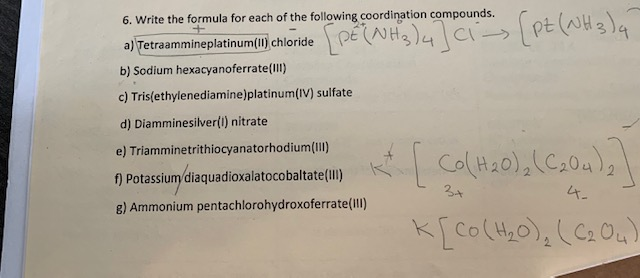PZ (WH3)4 6. Write the formula for each of the following coordination compounds. a) Tetraammineplatinum(II) chloride PE (NH3)4 Ci - b) Sodium hexacyanoferrate(III) c) Tris(ethylenediamine)platinum(IV) sulfate d) Diamminesilver(I) nitrate e) Triamminetrithiocyanatorhodium(III) f) Potassium diaquadioxalatocobaltate(Ill) g) Ammonium pentachlorohydroxoferrate(III) 3+ k[co (H₂O), (C2O4)

#### Homework Answers

Answer #1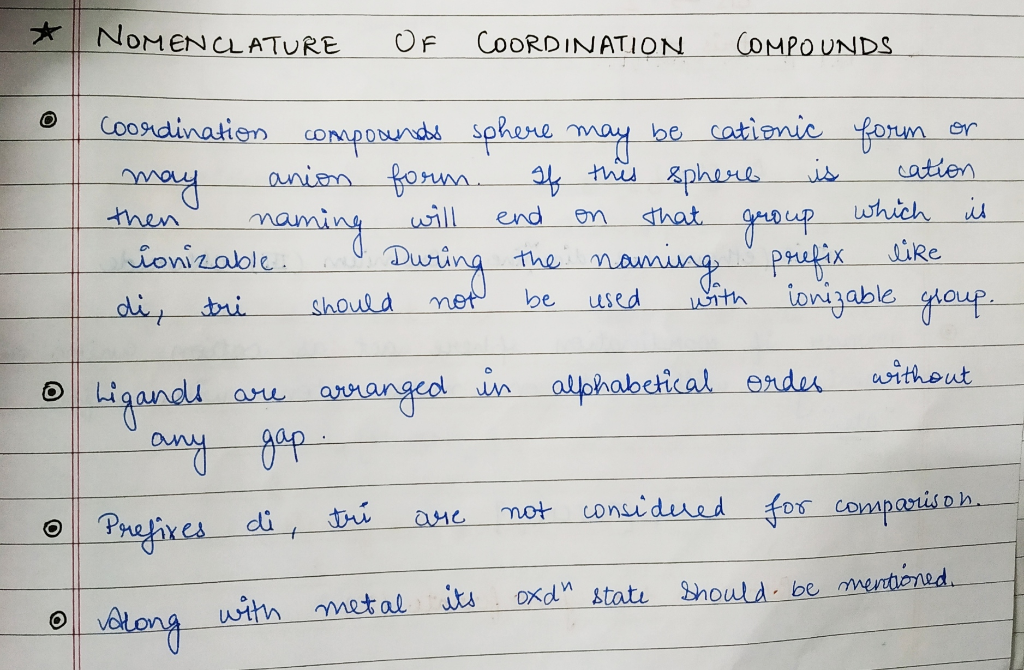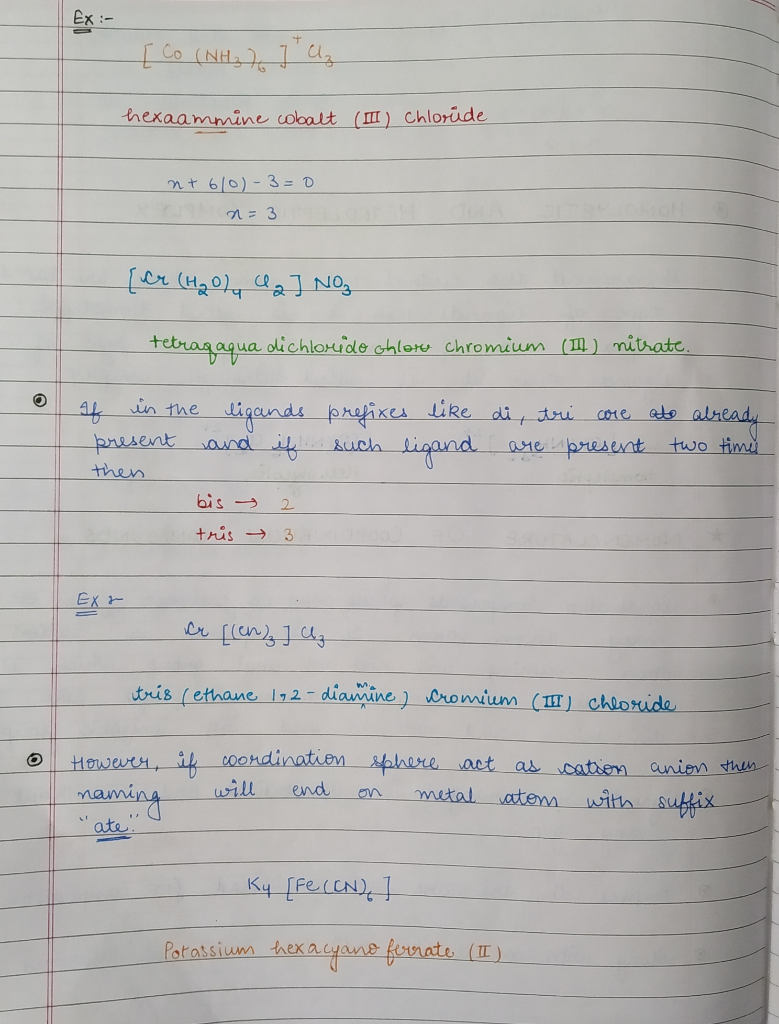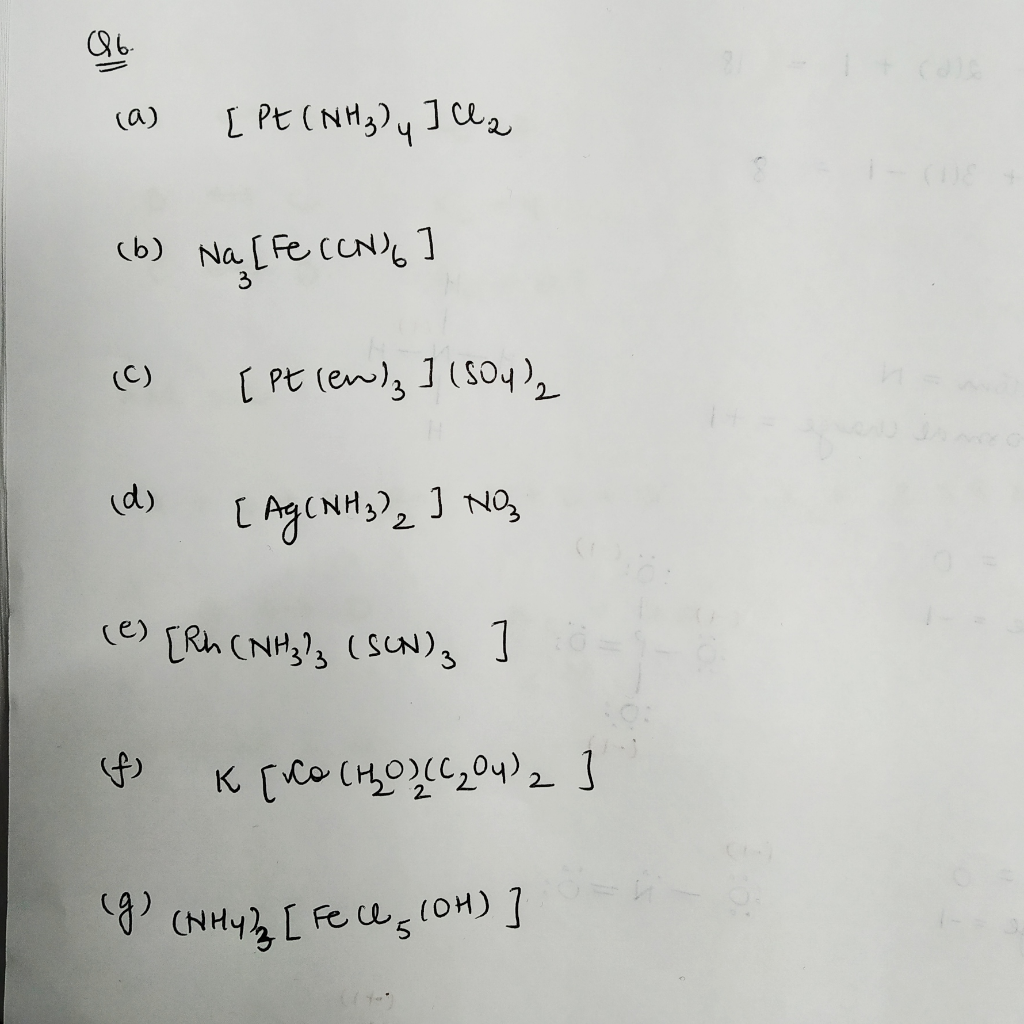Know the answer?
Your Answer:

#### Post as a guest

Your Name:

What's your source?

#### Earn Coin

Coins can be redeemed for fabulous gifts.

Not the answer you're looking for? Ask your own homework help question. Our experts will answer your question WITHIN MINUTES for Free.
Similar Homework Help Questions
• ### Coordination Compounds

Consider these six coordination compounds:A: NH4[Pt(NH3)Cl3]B: tris(ethylenediamine)cobalt(III) sulfateC: tetraaquadichloronickel(IV) chlorideD: [Co(NH3)2(en)Cl2]ClE: [Co(en)2Cl2]BrF: sodium hexacyanoferrate(II)(1) Dissolving one mole of salt in water yields six moles of ions. (Select all that apply.)A: NH4[Pt(NH3)Cl3]B: tris(ethylenediamine)cobalt(III) sulfateC: tetraaquadichloronickel(IV) chlorideD: [Co(NH3)2(en)Cl2]ClE: [Co(en)2Cl2]BrF: sodium hexacyanoferrate(II)None of these compounds(2) The transition metal ion has a coordination number of three. (Select all that apply.)A: NH4[Pt(NH3)Cl3]B: tris(ethylenediamine)cobalt(III) sulfateC: tetraaquadichloronickel(IV) chlorideD: [Co(NH3)2(en)Cl2]ClE: [Co(en)2Cl2]BrF: sodium hexacyanoferrate(II)None of these compounds(3) The coordination compound has four stereoisomers (geometric plus optical isomers). (Select all...

• ### LIFE SAVER!! Write formula for each compounds

Write the formula for each of the following compounds:a)Diamminesilver (I) nitrateb)Potassium diaquadioxalatocobaltate(III)c) Hexacarbonylmolybdenum(0)d) Diamminebis(ethylenediamine)chromium(III) chloride

• ### Write the correct formula for each complex ion or coordination compound

Write the correct formula for each complex ion or coordination compound.a) hexaaquanickle(II) chlorideb) pentacarbnoylchloromanganese(I)c) ammonium diaquatetrabromovanadate (III)d) tris (ethylenediamine)cobalt (III) trioxalatoferrate (III)

• ### Write formula for compounds wite the correct formula for the following compounds: sodium bromide calcium chloride...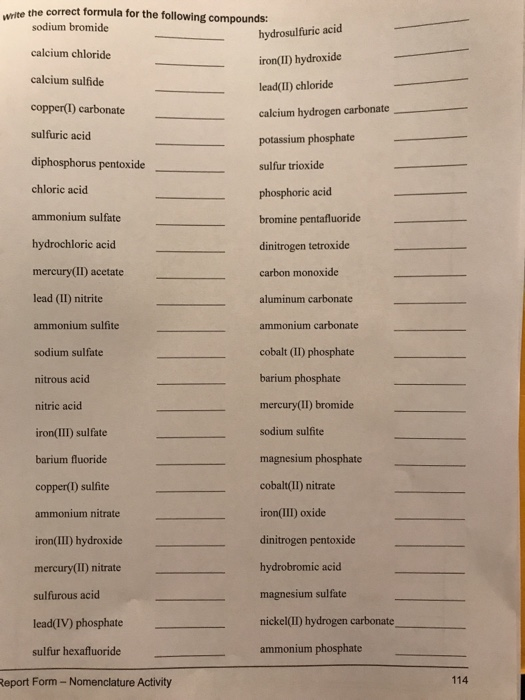Write formula for compounds wite the correct formula for the following compounds: sodium bromide calcium chloride calcium sulfide copper(I) carbonate sulfuric acid diphosphorus pentoxide _ chloric acicd ammonium sulfate hydrochloric acid mercury(II) acetate lead (II) nitrite ammonium sulfite sodium sulfate nitrous acid nitric acid iron(III) sulfate barium fluoride copper(I) sulfite ammonium nitrate iron(III) hydroxide mercury(II) nitrate sulfurous acid hydrosulfuric acid iron(II) hydroxide lead(II) chloride calcium hydrogen carbonate sulfur trioxide phosphoric acid bromine pentafluoride dinitrogen tetroxide carbon monoxide aluminum carbonate ammonium...

• ### Naming Compounds

Name each of the following compounds, being sure to use brackets to indicate the coordination sphere:A. hexamminechromium(III) nitrateB. tetraminecarbonatocobalt(III) sulfateC. dichlorobis(ethylenediamine)platinum(IV) bromideD. potassium diaquatetrabromovanadate(III)E. bis(ethylenediamine)zinc(II) tetraiodomercurate(II)

• ### Write the formula of the coordination compound tris(bipyridine)iron(II) bromide. Enclose complexes in square brackets, even if...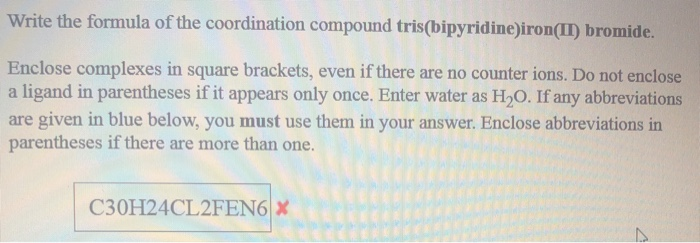Write the formula of the coordination compound tris(bipyridine)iron(II) bromide. Enclose complexes in square brackets, even if there are no counter ions. Do not enclose a ligand in parentheses if it appears only once. Enter water as H2O. If any abbreviations are given in blue below, you must use them in your answer. Enclose abbreviations in parentheses if there are more than one. C30H24CL2FEN6 X Name the coordination compound Li4[Zr(C204)4]. Give the chemical formula of the following coordination compound. Enclose complexes...

• ### O THE TRANSITION METALS Naming coordination compounds Write the chemical formula of these coordination compounds. formula...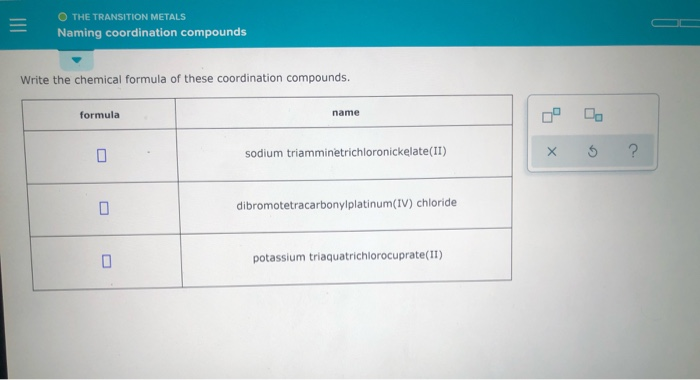O THE TRANSITION METALS Naming coordination compounds Write the chemical formula of these coordination compounds. formula name sodium triamminetrichloronickelate(II) x 6 ? dibromotetracarbonylplatinum(IV) chloride potassium triaquatrichlorocuprate(11)

• ### 1. 2. systematic name of : [Co(NH3)5Cl] Cl2 Give the formula of each coordination compound. Include...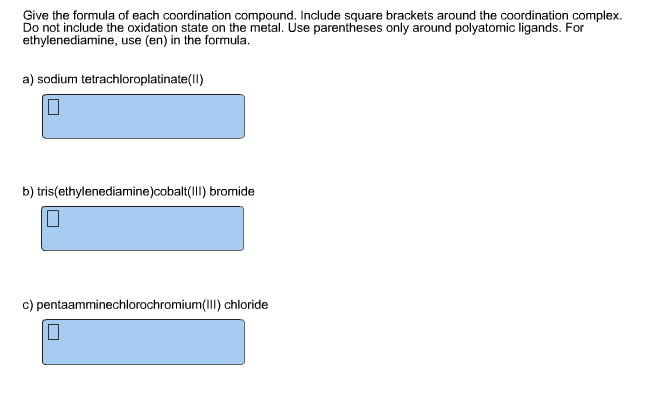1. 2. systematic name of : [Co(NH3)5Cl] Cl2 Give the formula of each coordination compound. Include square brackets around the coordination complex. Do not include the oxidation state on the metal. Use parentheses only around polyatomic ligands. For ethylenediamine, use (en) in the formula. a) sodium tetrachloroplatinate(II) b) tris(ethylenediaminbaI) bromide c) pentaamminechlorochromium(lIl) chloride

• ### Name or write the formula for the following coordination compounds (spelling counts): a. [Co(NH3)2Cl]Cl2 ______________________________ b....

Name or write the formula for the following coordination compounds (spelling counts): a. [Co(NH3)2Cl]Cl2 ______________________________ b. Sodium hexachloromanganate(III) _______________________ c. [Pt(NH3)4](ClO4)2 _____________________________________ d. Potassium tetracyanonickelate(0)

• ### Name or write the formula for the following coordination compounds (spelling counts): a. [Co(NH3)2CI]Cl2 b. Sodium...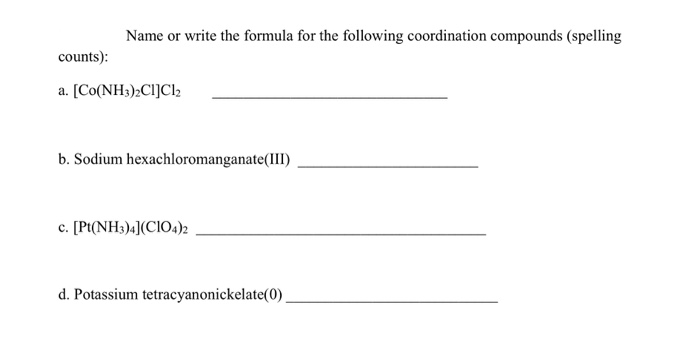Name or write the formula for the following coordination compounds (spelling counts): a. [Co(NH3)2CI]Cl2 b. Sodium hexachloromanganate(III) c. [Pt(NH3)4](C104)2 d. Potassium tetracyanonickelate(0)